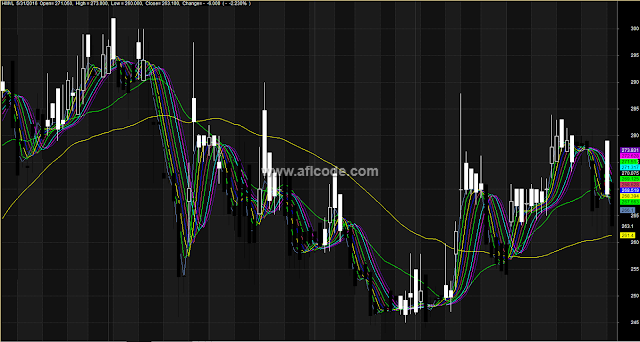Rainbow-Parabolic-Sar-Modified-Trading-System

```//www.aflcode.com
MaxGraph = 15;

Graph0 = Close;
Graph1 = MA(C,2);
Graph2 = MA(MA(C,2),2);
Graph3 = MA(MA(MA(C,2),2),2);
Graph4 = MA(MA(MA(MA(C,2),2),2),2);
Graph5 = MA(MA(MA(MA(MA(C,2),2),2),2),2);
Graph6 = MA(MA(MA(MA(MA(MA(C,2),2),2),2),2),2);
Graph7 = MA(MA(MA(MA(MA(MA(MA(C,2),2),2),2),2),2),2);
Graph8 = MA(MA(MA(MA(MA(MA(MA(MA(C,2),2),2),2),2),2),2),2);
Graph9 = MA(MA(MA(MA(MA(MA(MA(MA(MA(C,2),2),2),2),2),2),2),2),2);
Graph10 = MA(MA(MA(MA(MA(MA(MA(MA(MA(MA(C,2),2),2),2),2),2),2),2),2),2);

Graph0Style =64;
Graph1Style=Graph2Style=Graph3Style=Graph4Style=Graph5Style=Graph6Style=Graph7Style=Graph8Style=Graph9Style=Graph10Style = 1;
Graph0BarColor=IIf(SAR(0.05)<C,2,1);
Graph1Color = 3;
Graph2Color = 5;
Graph3Color =7;
Graph4Color = 6;
Graph5Color =15;
Graph6Color = 8;
Graph7Color =16;
Graph8Color = 10;
Graph9Color = 11;
Graph10Color = 12;
Graph11=MA(C,20);
Graph11Style=1;
Graph11Color=5;
Graph12=MA(C,50);
Graph12Style=1;
Graph12Color=7;

Title=Name() +"  "+Date()+"  Open="+WriteVal(O)+
",  High ="+WriteVal(H)+",  Low ="+WriteVal(L)+
",  Close="+WriteVal(C)+",  Change="+WriteIf((C-Ref(C,-1))>0," +"," -")+
WriteVal(C-Ref(C,-1))
+"  ("+WriteIf((C-Ref(C,-1))>0," +"," -")+WriteVal((C-Ref(C,-1))/Ref(C,-1)*100)+"%  )";
```
Previous
Next Post »